Next: Corrections to the probability Up: Basic principles of time Previous: Signal detection

Test statistics

The test statistic used for detection is a special case of a function of random variables. Testing the hypothesis Ho using the statistics S is a standard statistical procedure. Important examples of the test statistics are signal variances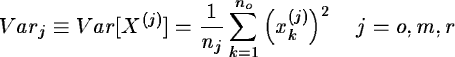(12.1)

For white noise (Ho is true), the distribution of Varj is a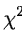distribution. It is remarkable that, then, Varm and Varr are statistically independent. Note further the inequality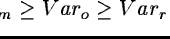which is due to the extra variance, Varm, in the model signal with respect to the variance, Varo, of the observations. The equality in these relations holds for pure noise.

The larger the variance of the model series Varm compared to the residual variance Varr is, the more significant is the detection or the better is the current parameter estimate, for problems (1) and (2) respectively (Sect. 12.2). Usually, the test statistics S in TSA measure a ratio of two variances. They differ according to the models assumed and the combination of the variances chosen. Since models depend on frequency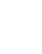(or time lag l), so do the variances Varm and Varr and test statistics S.

The statistics we recommend for use in the frequency domain are the ones introduced by Scargle and the Analysis of Variance (AOV) statistics. These statistics are used in the MIDAS commands SCARGLE/TSA, ORT/TSA and AOV/TSA (Sect. 12.4.6). The SCARGLE/TSA command uses a pure sine model, the ORT/TSA uses Fourier series and the AOV/TSA uses a step function (phase binning). In the time domain, we recommend to use thestatistic with the COVAR/TSA and DELAY/TSA commands (Sect. 12.4.7). Both COVAR/TSA and DELAY/TSA are based on a second series of observations which is used for the model. COVAR/TSA and DELAY/TSA differ in the method used for the interpolation of the series: the former deploys a step function (binning) while the latter relies on an analytical approximation of the autocorrelation function (ACF, Sect. 12.3.2) as a more elaborate approach. Among many other statistics we mention the one by Lafler & Kinman (1965), phase dispersion minimization (PDM) also known as the Whittaker & Robinson statistic (Stellingwerf, 1978), string length (Dvoretsky, 1983), and statistic introduced by Renson (1983).

In the limit of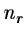(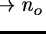) the sums of squares and degrees of freedom converge and so does the variance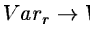(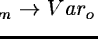). Since, increasing the number of parameters of a model nm to noimplies a decrease of Varm and a corresponding decrease in the significance of the detection. Therefore, we do not recommend to use models (e.g. long Fourier series, fine phase binning, string length and Renson statistics) with more parameters than are really required for the detection of the feature in question.

In the above limits, Varo and Varr (Varm) become perfectly correlated. Since all statistics named above except AOV use Varoat least implicitly, their probability distribution may, because of this correlation, differ considerably from what is generally supposed in the literature (Schwarzenberg-Czerny, 1989). However, the correlation vanishes in the asymptotic limit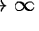for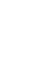, Scargle and Whitteker & Robinson statistics, so that they yield correct results for sufficiently large data sets. Please note that the problem of correlation aggravates for observations with high signal-to-noise ratio,, as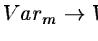, so that the statistics mentioned as using these variances become rather insensitive.Next: Corrections to the probability Up: Basic principles of time Previous: Signal detection
Petra Nass
1999-06-15